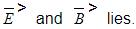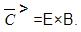Courses

# Test: Electromagnetic Waves

## 30 Questions MCQ Test Physics Class 12 | Test: Electromagnetic Waves

Description
This mock test of Test: Electromagnetic Waves for JEE helps you for every JEE entrance exam. This contains 30 Multiple Choice Questions for JEE Test: Electromagnetic Waves (mcq) to study with solutions a complete question bank. The solved questions answers in this Test: Electromagnetic Waves quiz give you a good mix of easy questions and tough questions. JEE students definitely take this Test: Electromagnetic Waves exercise for a better result in the exam. You can find other Test: Electromagnetic Waves extra questions, long questions & short questions for JEE on EduRev as well by searching above.
QUESTION: 1

### According to Maxwell’s equations

Solution:
*Multiple options can be correct
QUESTION: 2

### Electromagnetic waves for TV and radio have a frequency about

Solution:

Radio waves have frequencies as high as 300 gigahertz (GHz) to as low as 30 hertz (Hz). Like all other electromagnetic waves, radio waves travel at the speed of light in vacuum (and close to the speed of light in the Earth's atmosphere, which acts as the transmission media for the vast majority of terrestrial use).

QUESTION: 3

### Plane electromagnetic wave travels in vacuum along z-direction. If the frequency of the wave is 30 MHz, its wavelength is

Solution:

The electromagnetic wave travels in vacuum along z-direction. Then, the electric field vector and magnetic field vector will be in the XY plane.
Frequency of the wave = 30 MHz
Converting into Hz, v = 30× 106 Hz.
speed of light, c = 3 × 108 m/s
Wavelength of the wave can be calculated using the following relation:
λ = c/v
⇒ λ = 3 × 108/30 × 106
⇒λ = 10 m

QUESTION: 4

Electromagnetic waves propagate

Solution:

The frequency of radiation arising from the two close energy levels in hydrogen known as Lamb shift i.e. 1057 MHz is radio waves as it belongs to the short wavelength end of the electromagnetic spectrum.

QUESTION: 5

State the part of the electromagnetic spectrum to which 1057 MHz (frequency of radiation arising from two close energy levels in hydrogen; known as Lamb shift)..Belongs

Solution:

The frequency of radiation arising from the two close energy levels in hydrogen known as Lamb shift i.e. 1057 MHz is radio waves as it belongs to the short wavelength end of the electromagnetic spectrum. this wavelength correspond to microwave region of electromagnetic waves.

QUESTION: 6

Long distance radio broadcasts use short-wave bands because

Solution:

Long distance radio broadcasts use short-wave bands because they can be reflected by the ionosphere of the earth's atmosphere and thus can be send to longer distances.

QUESTION: 7

Which of these mechanisms can be used to produce electromagnetic waves?

Solution:

The electromagnetic waves are produced by vibration of an electric charge. This creates a wave which is both as electric and magnetic components. This way oscillates in perpendicular planes with respect to each other and in a phase.This creates all electromagnetic waves begin with an oscillating charged particle which creates an oscillating electric and magnetic field.

*Multiple options can be correct
QUESTION: 8

Microwaves have a frequency of about

Solution:

Microwave is a form of electromagnetic radiation with wavelengths ranging from about one meter to one millimeter; with frequencies between 300 MHz (1 m) and 300 GHz (1 mm). Different sources define different frequency ranges as microwaves; the above broad definition includes both UHF and EHF (millimeter wave) bands.
Answer B and D both are correct.

QUESTION: 9

The amplitude of the magnetic field part of a harmonic electromagnetic wave in vacuum is B0= 510 nT. Amplitude of the electric field part of the wave is

Solution:

Magnetic field part of a harmonic electromagnetic wave in vacuum
,B0​=510×10−9T
Speed of light,
C=3×108m/s
E=cBo​=153N/C

QUESTION: 10

It is necessary to use satellites for long distance TV transmission because

Solution:

TV signals being of high frequency are not reflected by the ionosphere. Therefore, to reflect these signals, satellites are needed. That is why, satellites are used for long distance TV transmission.
Most long-distance shortwave (high frequency) radio communication—between 3 and 30 MHz—is a result of skywave propagation.
This 3-30 MHz is a range of frequencies which are used in sky waves propagation so that the ionosphere is capable of reflecting it.

QUESTION: 11

The intensity of a plane electromagnetic wave is proportional to

Solution:

Long distance radio broadcasts use shortwave bands because only these bands can be refracted by the ionosphere.

QUESTION: 12

According to Maxwell’s equations

Solution:

Maxwell’s Fourth Equation
It is based on Ampere’s circuital law. To understand Maxwell’s fourth equation it is crucial to understand Ampere’s circuital law,
Consider a wire of current-carrying conductor with the current I, since there is an electric field there has to be a magnetic field vector around it. Ampere’s circuit law states that “The closed line integral of magnetic field vector is always equal to the total amount of scalar electric field enclosed within the path of any shape” which means the current flowing along the wire(which is a scalar quantity) is equal to the magnetic field vector (which is a vector quantity)

QUESTION: 13

The direction of propagation of an electromagnetic plane wave is

Solution:

The direction of propagation of the electromagnetic wave is always perpendicular to the plane in whichSo, the direction of the propagation of the wave,Hence, option B is the correct answer.

QUESTION: 14

Infrared waves are produced by

Solution:

Infrared waves are emitted by hot bodies. They are produced due to the de-excitation of atoms.
They are called Heat waves as they produce heat falling on matter. This is because water molecules present in most materials readily absorb infrared waves. After absorption, their thermal motion increases, that is, they heat up and heat their surroundings.
Uses: Infra red lamps; play an important role in maintaining warmth through greenhouse effect.

QUESTION: 15

Use the formula λm T = 0.29 cmK to obtain the characteristic temperature range for λm=5×10-7m

Solution:

A body at temperature T produces a continuous spectrum of wavelengths. For a black body, the wavelength corresponding to maximum intensity of radiation is given according to Planck's law by the relation, m=0.29cmK/T. For m=106m,T=2900K.Temperatures for other wavelengths can be found. These numbers tell us the temperature ranges required for obtaining radiations in different parts of the electromagnetic spectrum. Thus, to obtain visible radiation, say  =5×107m, the source should have a temperature of about 6000K. A lower temperature will also produce this wavelength but not the maximum intensity.

QUESTION: 16

Optical and radio telescopes are built on the ground, but X-ray Astronomy is possible only from satellites orbiting the earth because

Solution:

X-rays are absorbed by the atmosphere and therefore the source of X-rays must lie outside the atmosphere to carry out X-ray astronomy and therefore satellites orbiting the earth are necessary but radio waves and visible light can penetrate through the atmosphere and therefore optical and radio telescopes can be built on the ground.

QUESTION: 17

Plane electromagnetic waves are

Solution:

E is the electric field vector, and B is the magnetic field vector of the EM wave. For electromagnetic waves E and B are always perpendicular to each other and perpendicular to the direction of propagation. Electromagnetic waves are transverse waves. The wave number is k = 2π/λ, where λ is the wavelength of the wave.

QUESTION: 18

Without the concept of displacement current it is not possible to correctly apply Ampere’s law on a path parallel to the plates of parallel plate capacitor C in

Solution:
QUESTION: 19

Visible range of electromagnetic spectrum is

Solution:

The visible light spectrum is the segment of the electromagnetic spectrum that the human eye can view. More simply, this range of wavelengths is called visible light. Typically, the human eye can detect wavelengths from 380 to 700 nanometers.
Option C is the closest so the answer is option C.

QUESTION: 20

State the part of the electromagnetic spectrum to which 21 cm (wavelength emitted by atomic hydrogen in interstellar space).belongs

Solution:

Radio waves have wavelengths of 1mm to 100km. Hence, 21cm lies at short wavelength end of radio waves.

QUESTION: 21

Velocity of plane electromagnetic waves in vacuum equals

Solution:

The speed of any electromagnetic waves in free space is the speed of light c = 3x108 m/s. Electromagnetic waves can have any wavelength λ or frequency f as long as λf = c.

QUESTION: 22

Tanning of the skin in sunlight is due to

Solution:

UVA radiation is what makes people tan. UVA rays penetrate to the lower layers of the epidermis, where they trigger cells called melanocytes (pronounced: mel-an-oh-sites) to produce melanin. Melanin is the brown pigment that causes tanning. Melanin is the body's way of protecting skin from burning.

QUESTION: 23

A charged particle oscillates about its mean equilibrium position with a frequency of 109 Hz. Frequency of the electromagnetic waves produced by the oscillator is

Solution:

The frequency of an electromagnetic wave produced by the oscillator is same as that of a charged particle oscillating about its mean position i.e., 109Hz.

QUESTION: 24

If the earth did not have an atmosphere, would its average surface temperature be higher or lower than what it is now?

Solution:

The radiation of infrared rays of the sun keeps the earth's surface warm even at night due to the greenhouse effect of the atmosphere. Heavy gases likely CO2​ present in the earth's atmosphere reflect infra-red radiation back towards the earth's surface. Due to which earth's atmosphere becomes richer in infrared radiation. If the atmosphere is absent then earth temperature falls off.

QUESTION: 25

Do EM waves need a medium to travel through?

Solution:

The electromagnetic waves are not mechanical waves. There are vibrations of electric vectors and magnetic vectors in them. These vibrations do not need any particles present in the medium for their propagation. That's why electromagnetic waves do not require any medium for propagation.This means that electromagnetic waves can travel not only through air and solid materials, but also through the vacuum of space.

QUESTION: 26

Comparing X-rays and Gamma rays

Solution:

Gamma-ray photons have the highest energy in the EMR spectrum and their waves have the shortest wavelength. Scientists measure the energy of photons in electron volts (eV). X-ray photons have energies in the range 100 eV to 100,000 eV (or 100 keV). Gamma-ray photons generally have energies greater than 100 keV.

QUESTION: 27

7.5 MHz to 12 MHz band corresponds to wavelength band of

Solution:

f​1=7.5×106 Hz
f2​=12×106 Hz
λ1​=c/f1​=40 m
λ2​=c/f2​=25 m

So, the range is 40m to 25m

QUESTION: 28

The speed of plane electromagnetic waves is maximum in

Solution:

The speed of any electromagnetic waves in free space is the speed of light c = 3x108 m/s. Electromagnetic waves can have any wavelength λ or frequency f as long as λf = c.

QUESTION: 29

When white light is split into its component colors by a prism

Solution:

when white light enters the prism it has actually entered out atmosphere and so the parts of the EM spectrum like UV don't appear. After the light comes out of the prism all the parts separate but we can only see the visible part i.e. 400-700nm range. So we look at only a part of the EM spectrum. Hence option B is correct.

QUESTION: 30

Some scientists have predicted that a global nuclear war on the earth would be followed by a severe ‘nuclear winter’ with a devastating effect on life on earth. What might be the basis of this prediction?

Solution:

The use of nuclear weapons would cause the formation of smoke clouds preventing the light from the sun reaching earth surface and it will also deplete the atmosphere and therefore stopping the greenhouse effect and thus doubling the cooling effect.
Hence the correct answer is option C.